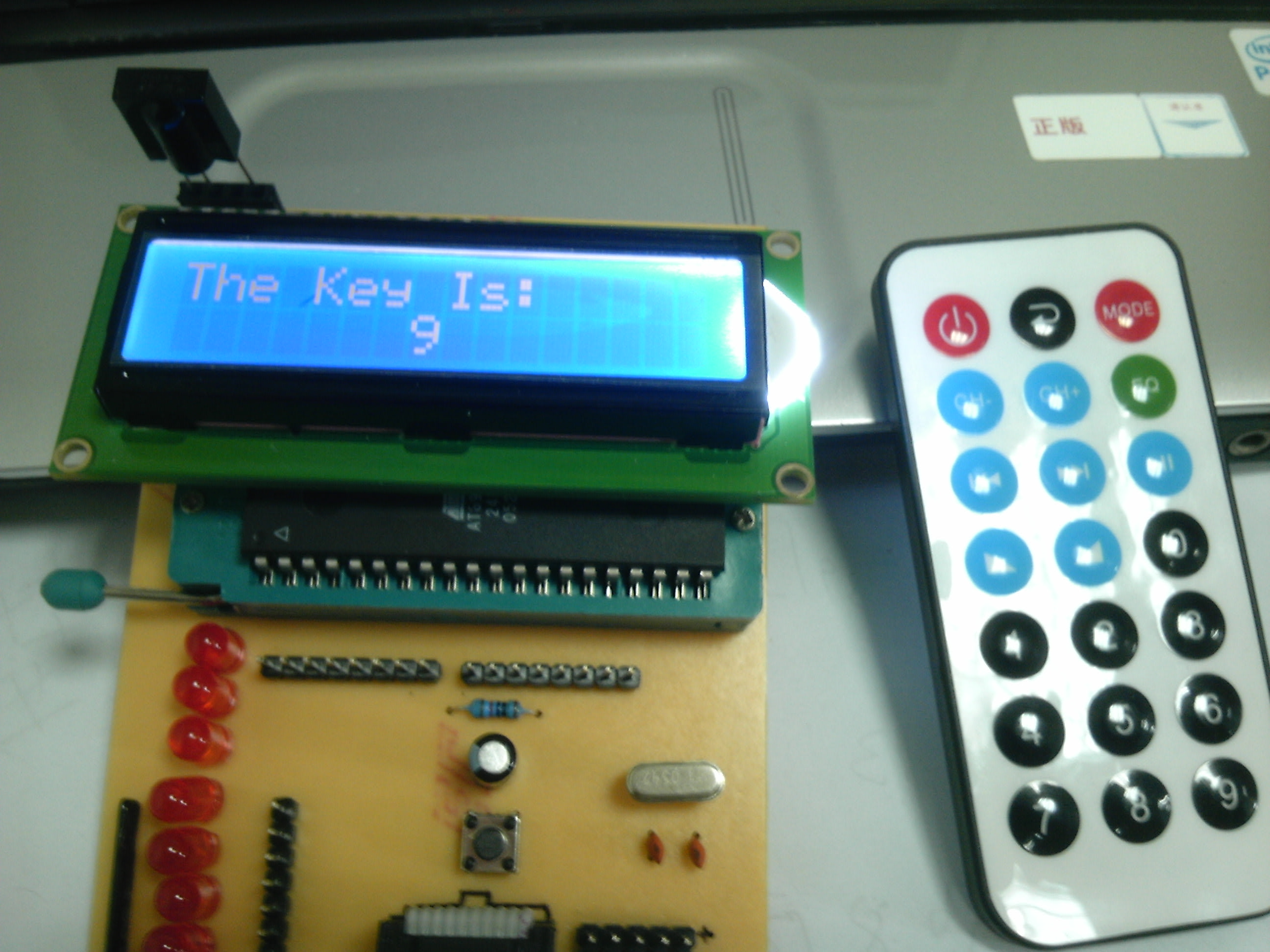# 单片机红外接收软件解码

HS0038 封装：                                                    VS838封装：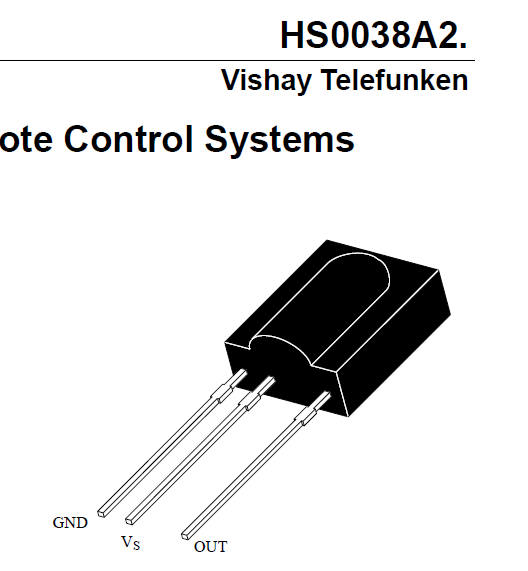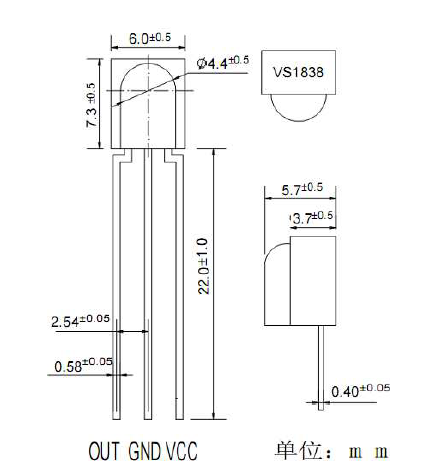NEC 标准：

一个完整的全码=引导码+用户码+用户码+数据码+数据反码。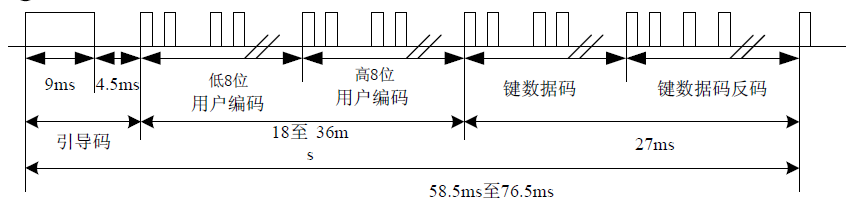NEC 标准下的发射码表示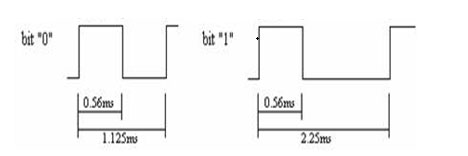PHILIPS 标准：

一个全码＝起始码‘11’＋控制码＋用户码+用户码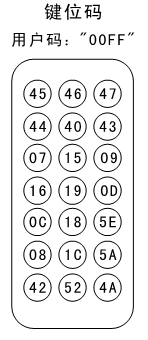`    #include <reg52.h>    #include “lcd1602.h”    #define uchar unsigned  char     // 宏定义uchar 为无符号字符    #define uint  unsigned  int    #define IR_UserCode 0xFF00            //红外遥控器 用户码       uchar IR_Code = {0};    uchar IR_User = {0};  //用户码    uchar IR_Data  = {0};  //数据码    uchar IR_CData = {0};  //数据反码    uchar key= ‘0’,value = 0; //key LCD显示值; value  接收头接收到值（16进制）    typedef enum    {        State_0 = 0x00,        State_1 = 0x01,        State_2 = 0x02    }ScanState_Typedef;     ScanState_Typedef ScanState;    void  IR_Check(void);    void  IR_CodeHandle(void);    void  IR_Decode(void);    void  delay(uint n);    void main()    {        LCD_Init();        LCD_WriteString(“The Key Is:”,1);        while(1){            IR_Check();        }    }    void  IR_Check(void)    {        uchar i=0,n=0;        switch(ScanState)        {            case State_0 :                //检测引导码            {                while(IR_DQ == 1);                delay(500);                if( IR_DQ == 0 )                {                    while(IR_DQ == 0);                    delay(300);                    if(IR_DQ == 1)                    {                        ScanState = State_1;                        while( IR_DQ == 1 );                    }else{                        ScanState = State_2;        //为连发码                    }                }else{                         ScanState = State_0;                }                break;            }            case State_1:                       // 32位数据接收            {                while(i < 32){                    while(IR_DQ == 0);                    delay(60);                  //延时0.48ms                    if(IR_DQ == 1)                    IR_Code[i] = 0;                    delay(28);                    //延时0.22ms,总延时0.70ms                    if(IR_DQ == 1)                    {                        IR_Code[i] = 1;                        while(IR_DQ == 1);                    }else{                        IR_Code[i] = 0;                    }                    i++;                }                IR_CodeHandle();                ScanState = State_0;                break;            }            case State_2:                        //连发码处理            {                while( IR_DQ == 0 );                ScanState = State_0;                break;            }        }    }    void IR_CodeHandle(void)    {        uchar n=0,check=0;        uint  user = 0;        for(n=0;n<8;n++)        {            IR_User[n] = IR_Code[n];      //低位用户码            IR_User[8+n] = IR_Code[8+n];  //高位用户码            IR_Data[n] = IR_Code[16+n];            IR_CData[n] = IR_Code[24+n];            if(IR_Data[n] + IR_CData[n] == 0x01){ check++;}       //反码检测,正数的原码和补码 各位相加后为 0x01        }        for(n=0;n<16;n++)                       //处理用户码        {            if(n==0)            {                user = IR_User;            }else{                user += IR_User[n]  (2 << (n-1));            }        }        if((user == IR_UserCode) && (check == 8))       //用户码校验 反码校验        {            for(n=0;n<8;n++)                   //处理数据码,二进制转换为16进制            {                if(n==0)                {                    value = IR_Data;                }else{                    value += IR_Data[n]  (2 << (n-1));                }            }            IR_Decode();            LCD_WriteByte(0xC7,0);           //更新接收值            LCD_WriteByte(key,1);        }    }    void  IR_Decode(void)    {        //P3 = value;        switch(value)        {            case 0x0D : key = ‘0’;break;            case 0x0C : key = ‘1’;break;            case 0x18 : key = ‘2’;break;            case 0x5E : key = ‘3’;break;            case 0x08 : key = ‘4’;break;            case 0x1C : key = ‘5’;break;            case 0x5A : key = ‘6’;break;            case 0x42 : key = ‘7’;break;            case 0x52 : key = ‘8’;break;            case 0x4A : key = ‘9’;break;            default : key= ‘?’;        }    }    void delay(uint n)    {        while(–n);                // 8us一次    }`
lcd1602.h 的程序见：  LCD显示汉字 一文